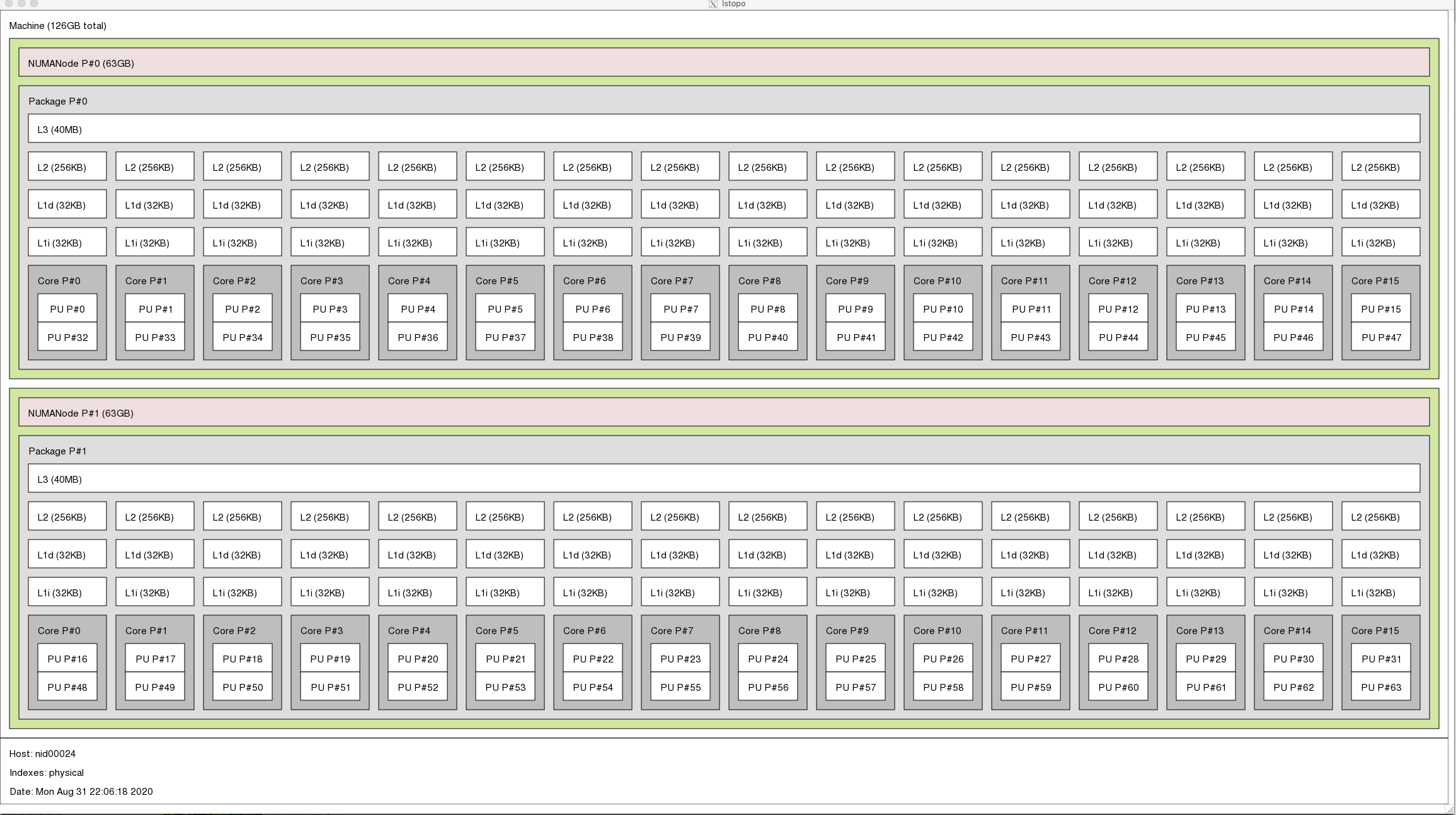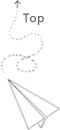# tips for numa and hyperthreading

there are some confusion about the NUMA and hyperthreading when execute the multi processes/threads testing or evaluation on clusters, take some notes here.

For the slurm, one core refer to one logical cpu core. For example in this documentation:

srun option “-c”: Set the value as “number of logical cores (CPUs) per MPI task” for MPI and hybrid MPI/OpenMP jobs. The “-c” flag is optional for fully packed pure MPI jobs.

If there is one physical cpu but there are two hyperthreading, there are two cores for slurm schduler. The interesting metaphors about the hyperthreading is cashier desk in canteen， if there is one line and one faculty set here to process the check for every one, this is one physical cpu and one logical cpu. If there are two lines and one faculaty sit in the middle, it looks that two people can be processes concurently, this is called one physical cpu with two hyperthreading (two logical cpu)

CPU and processor

Sometims, it is easy to misuse the term CPU, this answer provides some good explanation. Basically, there is 1:1 relationship between the concept of socket and the processor. One processor could contains one or more physical CPU, one physical CPU can contains 2 hyperthreading, or locaical CPUs. From the software’s perspective, one thread is running on one logical CPU at the same time slice. One process contains at lease one thread (system level thread).

vCPU and pCPU

If there is virtulization techniques, such as we create the virtual machine, the vCPU is the virtulized cpu for the VM, the pCPU is the actual hyperthreading cpu. There are lots of articles about the vCPU/pCPU ratio which is out of the scope of this article. generally speaking, we could map on vCPU into one pCPU. such as the explanation in this article.

### NUMA

There concepts of NUMA comes from the UMA, this is more discuss how cpu access the memory. This article provides a good history about the NUMA and UMA. In my opinion, one reason to move from the UMA to NUMA is the scalability, if all the cpu access the memory by same bus, it might slow down the averagy memory access time in large scale. The proper number of the subdomain (numa domain) can decrease the average memory access time in large scale. If there are only one numa domain, there is no pbvious difference compared wih the uniform memory access. For exmple, there is only one numa domain for my laptop:

There are 4 physical cores. The PU here represents the hyperthreading. For every physical core, they have separate L2 and L1 cache, but these cache are shared by the hyperthreading such as the PU listed under the core. For different cores, they share the L3 cache and the NUMA memory.

### scheduler’s perspective

one typical tool is the lstopo that shows the topology for the current node. For the parallel computing code, if the user hope to use the cache size wisely, it is important to use this tools to check the cache size and make the data block to match with the cache size.

The command numactl -H can be used to show the numa domain

For the large scale testing on specific cluster or supercomputer, the configuration is important, this is a good article on cori cluster about the affinity. There are sevearal levels, the first is the node level, which means that you may run tests several time and each time, the tasks are running on machines with same type of configurations. Mayber the partition parameter is important here.

Here is an exmaple of the node topology for a cluster node that we used in this parts.bind to cores

For example, one specific cluster. there are following commands can be used to check the core affinity:

If we use all cpus, the task are evenly distributed among two different numa domains.

If the cores per task (-c) is not a good number, the task will occupy two numa domains. If we do not use the cpu bind parameters, there are:

we could see that all cores are occupied even if there are only part of the cores are actually used for the second case (the process number is not a divisor of 64).

bind to numa domains

From the user’s perspective, bind the program to the numa domain equals to binding the process with the cores associated with this numa domain. If we start 4 process, each process allocates 8 cores, and use 32 cores in total. For the current node, we could set the 4 processes to the numa node 1.

If we use the following commands:

We could see that the slurm scheduler use the two different numa domains, obviously, it wants to provide some load balance between different numa domains. But this is not the case we wanted, and we hope to start these processes in one numa domain.

Although there are several options in --cpu-bind, it seems there is no one that can satisfy our requirments (i’m not sure how to do it yet), the only approximate one is this:

in this case, the processes are allocated into the same numa domain cpus but the granularity is the domain level instead of the cpu level.

For the ideal case: